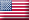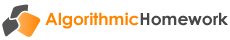Introduces the fundamental mathematical tools that are used in finance and builds a working knowledge of basic business calculations by providing unlimited practice of real world applications. Complexity of the mathematical formulas is minimised by the use of financial tables. This e-workbook is suitable for students with no previous experience of financial transactions or the mathematics that underlie them.

#### Fundamental concepts

• Concepts of risk and return
• The time value of money
• Positioning of cash flows on a timeline

#### Simple interest

• Calculating the amount of simple interest
• Calculating the number of days between dates
• Future value at simple interest
• Present value at simple interest
• Finding the interest rate and time period
• Promissory notes at simple interest

#### Compound interest

• Nominal, effective and equivalent rates
• Future value at compound interest
• Present value at compound interest
• Finding the interest rate and time period
• Equations of value

#### Annuities

• Future value of an ordinary annuity
• Present value of an ordinary annuity
• Present value of a deferred annuity
• Present value of a perpetuity
• Finding the periodic payment

#### Applications to personal finance

• Finding the periodic payment for a housing loan
• Calculating a loan repayment schedule
• Applications of business mathematics to bank accounts and credit cards

#### Applications to corporate finance

• Pricing a bond on a coupon date
• Making investment decisions based upon net present value
• Share valuation